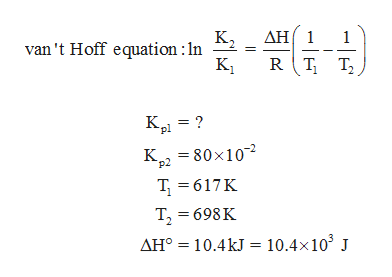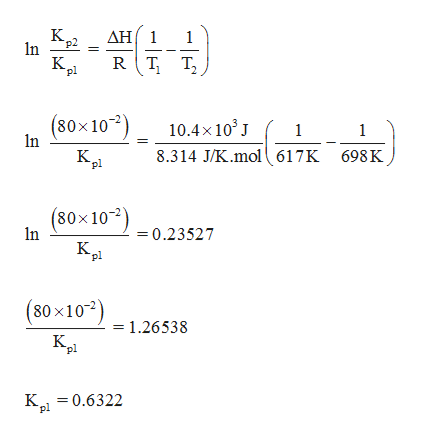The equilibrium constant, Kp, for the following reaction is 80×10-2at 698 K.2HI(g) H2(g) +  I2(g) If ΔH° for this reaction is 10.4 kJ, what is the value of Kp at 617 K?Kp =

Question
1. The equilibrium constant, Kp, for the following reaction is 80×10-2at 698 K.

2HI(g) H2(g) +  I2(g)

If ΔH° for this reaction is 10.4 kJ, what is the value of Kp at 617 K?

Kp =
Step 1

Kp at 617 K for the given reaction can be determined using van't Hoff equation.help_outlineImage TranscriptioncloseAH1 K2 van 't Hoff equation :ln K1 1 pl K22 80x10 T617K T 698K AH°10.4kJ= 10.4x103 J fullscreen
Step 2

Calculate Kp at 61...help_outlineImage TranscriptioncloseAH1 K p2 n 1 RT K pl T2 (80x102) 10.4x103 1 1 n 8.314 J/K.mol ( 617K K pl 698K (80x100.23527 In K "pl (80x101.26538 K K=0.6322 "pl fullscreen

Want to see the full answer?

See Solution

Want to see this answer and more?

Our solutions are written by experts, many with advanced degrees, and available 24/7

See Solution
Tagged in

Chemistry